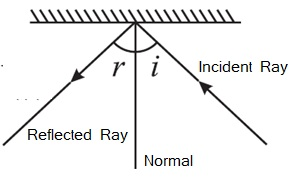Study Materials

# NCERT Solutions for Class 8th Science

Page 4 of 5

## Chapter 16. Light

Ques: What is incident ray?

Ans: The light ray, which strikes any surface, is called the incident ray.

Ques: What is reflected ray?

Ans: The ray that comes back from the surface after reflection is known as the reflected ray.Ques: Write the law of reflection.

Ans:

(i) Angle of incidence is always equal to the angle of reflection.

(ii) The incidence ray, the normal at the point of incidence and the reflected ray all lie in the same plane.

Ques: What is diffused or irregular reflection?

Ans: When all parallel rays reflected from a plane surface are not parallel, the reflection is known as  diffused or irregular reflection.

Ques: What is regular reflection?

Ans: Reflection from a smooth surface like that of a mirror is called regular reflection.

Ques: What is called the phenomenon, in an image formed by a mirror the left of the object appears on the right and the right appears on the left?

Ans: This phenomenon is called lateral inversion.

Ques: Write the use of Periscopes.

Ans: Periscopes are used in submarines, tanks and also by soldiers in bunkers to see things outside.

Ques: What is Kaleidoscope? Write its one use.

Ans: Kaleidoscope is an idea or a device that is made to combine the mirrors at an angle to one another to get number of images. It is used to make numerous beautiful patterns.

Ques: Write the nature of image of plane mirror.

Ans: The nature of image of plane mirror is real, behind the mirror and equal to object.

Ques: What is the angle of incidence of a ray if the reflected ray is at an angle of 90° to the incident ray?

Ans: 45o

.Sol : AOB = 90o

While

AOB = 90o

∠i + r = 90o

i + i = 90o     (i = r)

⇒2i = 90o

i = 90o/2

i = 45o

Ques: what is the colour of sun light?

Ans: White

Ques: What is dispersion of light?

Ans: Splitting of light into its colours is known as dispersion of light.

Ques: How does white sunlight look while passing through prism?

Ans: It looks like seven colour of rainbow.

Ques: Name a natural phenomenon to show the splitting of light.

Ans: Formation of rainbow.

Ques:What  is retina? Write its function?

Ans: The lens focuses light on the back of the eye, on a layer called retina.

Function of retina;

(i) Images are formed on retina.

Page 4 of 5

Chapter Contents: